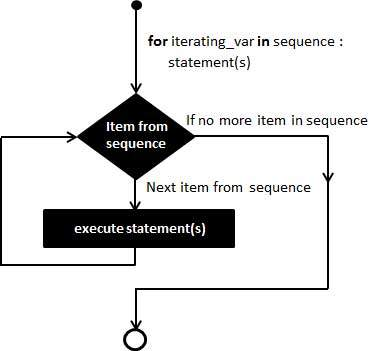# Python 3 - for Loop Statements

The for statement in Python has the ability to iterate over the items of any sequence, such as a list or a string.

### Syntax

```for iterating_var in sequence:
statements(s)
```

If a sequence contains an expression list, it is evaluated first. Then, the first item in the sequence is assigned to the iterating variable iterating_var. Next, the statements block is executed. Each item in the list is assigned to iterating_var, and the statement(s) block is executed until the entire sequence is exhausted.

### Flow Diagram## The range() function

The built-in function range() is the right function to iterate over a sequence of numbers. It generates an iterator of arithmetic progressions.

### Example

```>>> range(5)
range(0, 5)
>>> list(range(5))
[0, 1, 2, 3, 4]
```

### Example

range() generates an iterator to progress integers starting with 0 upto n-1. To obtain a list object of the sequence, it is typecasted to list(). Now this list can be iterated using the for statement.

```>>> for var in list(range(5)):
print (var)
```

### Output

This will produce the following output.

```0
1
2
3
4
```

### Example

```#!/usr/bin/python3

for letter in 'Python':     # traversal of a string sequence
print ('Current Letter :', letter)
print()
fruits = ['banana', 'apple',  'mango']

for fruit in fruits:        # traversal of List sequence
print ('Current fruit :', fruit)

print ("Good bye!")
```

### Output

When the above code is executed, it produces the following result −

```Current Letter : P
Current Letter : y
Current Letter : t
Current Letter : h
Current Letter : o
Current Letter : n

Current fruit : banana
Current fruit : apple
Current fruit : mango
Good bye!
```

## Iterating by Sequence Index

An alternative way of iterating through each item is by index offset into the sequence itself. Following is a simple example −

### Example

```#!/usr/bin/python3

fruits = ['banana', 'apple',  'mango']
for index in range(len(fruits)):
print ('Current fruit :', fruits[index])

print ("Good bye!")
```

### Output

When the above code is executed, it produces the following result −

```Current fruit : banana
Current fruit : apple
Current fruit : mango
Good bye!
```

Here, we took the assistance of the len() built-in function, which provides the total number of elements in the tuple as well as the range() built-in function to give us the actual sequence to iterate over.

## Using else Statement with Loops

Python supports having an else statement associated with a loop statement.

• If the else statement is used with a for loop, the else block is executed only if for loops terminates normally (and not by encountering break statement).

• If the else statement is used with a while loop, the else statement is executed when the condition becomes false.

### Example

The following example illustrates the combination of an else statement with a for statement that searches for even number in given list.

```#!/usr/bin/python3

numbers = [11,33,55,39,55,75,37,21,23,41,13]

for num in numbers:
if num%2 == 0:
print ('the list contains an even number')
break
else:
print ('the list doesnot contain even number')
```

### Output

When the above code is executed, it produces the following result −

```the list does not contain even number
```
python_loops.htm